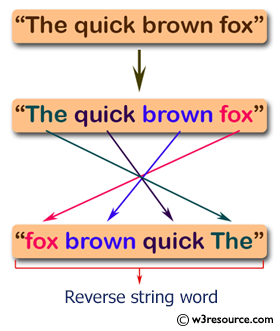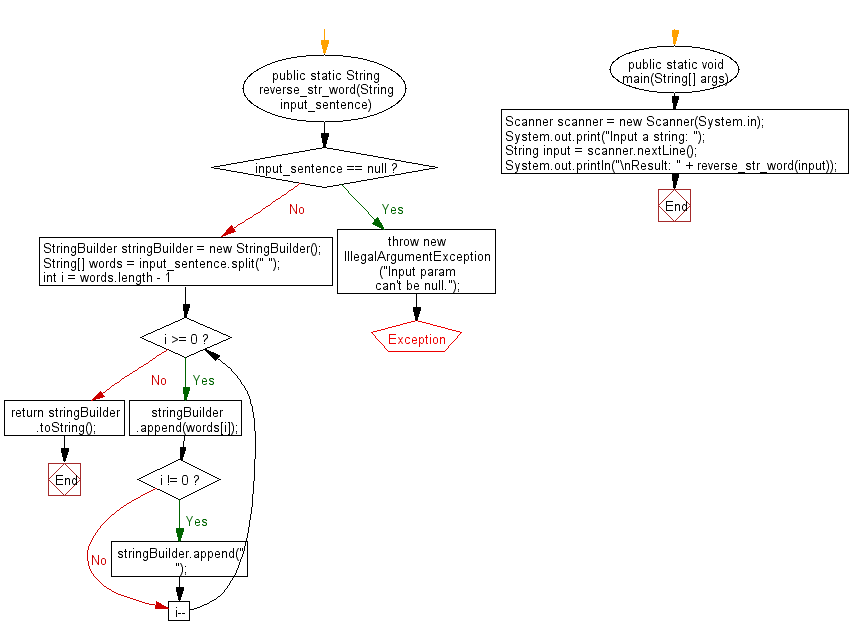﻿ Java: Reverse a sentence without reverse every word

# Java Exercises: Reverse the content of a sentence without reverse every word

## Java Basic: Exercise-169 with Solution

Write a Java program to reverse the content of a sentence (assume a single space between two words) without reverse every word.

Pictorial Presentation:Sample Solution:

Java Code:

``````import java.util.*;
public class Solution {
public static String reverse_str_word(String input_sentence) {
if (input_sentence == null) {
throw new IllegalArgumentException("Input param can't be null.");
}
StringBuilder stringBuilder = new StringBuilder();
String[] words = input_sentence.split(" ");
for (int i = words.length - 1; i >= 0; i--) {
stringBuilder.append(words[i]);
if (i != 0) {
stringBuilder.append(" ");
}
}
return stringBuilder.toString();
}
public static void main(String[] args) {
Scanner scanner = new Scanner(System.in);
System.out.print("Input a string: ");
String input = scanner.nextLine();
System.out.println("\nResult: " + reverse_str_word(input));
}
}
```
```

Sample Output:

```Input a string:  The quick brown fox jumps over the lazy dog

Result: dog lazy the over jumps fox brown quick The
```

Flowchart:Java Code Editor:

What is the difficulty level of this exercise?

Test your Programming skills with w3resource's quiz.

﻿

## Java: Tips of the Day

Java: Anagrams

Generates all anagrams of a string.

```public static List<String> anagrams(String input) {
if (input.length() <= 2) {
return input.length() == 2
? Arrays.asList(input, input.substring(1) + input.substring(0, 1))
: Collections.singletonList(input);
}
return IntStream.range(0, input.length())
.mapToObj(i -> new SimpleEntry<>(i, input.substring(i, i + 1)))
.flatMap(entry ->
anagrams(input.substring(0, entry.getKey()) + input.substring(entry.getKey() + 1))
.stream()
.map(s -> entry.getValue() + s))
.collect(Collectors.toList());
}
```